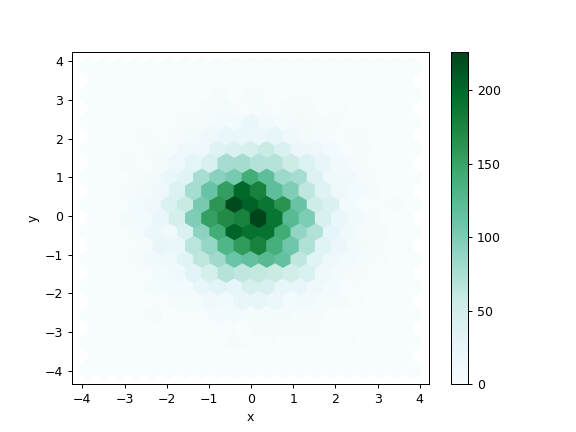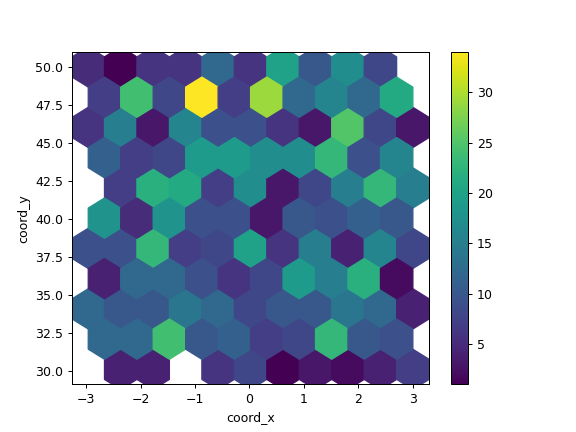# pandas.DataFrame.plot.hexbin¶

DataFrame.plot.hexbin(self, x, y, C=None, reduce_C_function=None, gridsize=None, **kwargs)[source]

Generate a hexagonal binning plot.

Generate a hexagonal binning plot of x versus y. If C is None (the default), this is a histogram of the number of occurrences of the observations at (x[i], y[i]).

If C is specified, specifies values at given coordinates (x[i], y[i]). These values are accumulated for each hexagonal bin and then reduced according to reduce_C_function, having as default the NumPy’s mean function (numpy.mean()). (If C is specified, it must also be a 1-D sequence of the same length as x and y, or a column label.)

Parameters
xint or str

The column label or position for x points.

yint or str

The column label or position for y points.

Cint or str, optional

The column label or position for the value of (x, y) point.

reduce_C_functioncallable, default np.mean

Function of one argument that reduces all the values in a bin to a single number (e.g. np.mean, np.max, np.sum, np.std).

gridsizeint or tuple of (int, int), default 100

The number of hexagons in the x-direction. The corresponding number of hexagons in the y-direction is chosen in a way that the hexagons are approximately regular. Alternatively, gridsize can be a tuple with two elements specifying the number of hexagons in the x-direction and the y-direction.

**kwargs

Additional keyword arguments are documented in DataFrame.plot().

Returns
matplotlib.AxesSubplot

The matplotlib Axes on which the hexbin is plotted.

DataFrame.plot

Make plots of a DataFrame.

matplotlib.pyplot.hexbin

Hexagonal binning plot using matplotlib, the matplotlib function that is used under the hood.

Examples

The following examples are generated with random data from a normal distribution.

>>> n = 10000
>>> df = pd.DataFrame({'x': np.random.randn(n),
...                    'y': np.random.randn(n)})
>>> ax = df.plot.hexbin(x='x', y='y', gridsize=20)The next example uses C and np.sum as reduce_C_function. Note that ‘observations’ values ranges from 1 to 5 but the result plot shows values up to more than 25. This is because of the reduce_C_function.

>>> n = 500
>>> df = pd.DataFrame({
...     'coord_x': np.random.uniform(-3, 3, size=n),
...     'coord_y': np.random.uniform(30, 50, size=n),
...     'observations': np.random.randint(1,5, size=n)
...     })
>>> ax = df.plot.hexbin(x='coord_x',
...                     y='coord_y',
...                     C='observations',
...                     reduce_C_function=np.sum,
...                     gridsize=10,
...                     cmap="viridis")